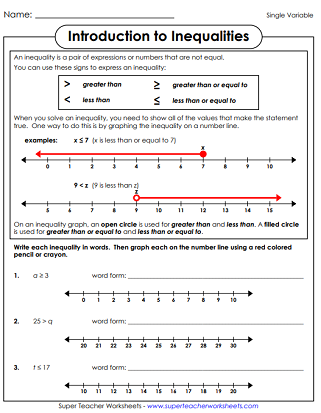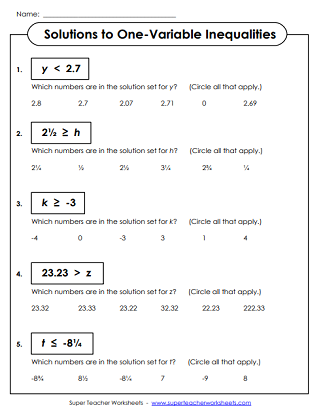# Single Variable Inequalities

On each of these printable pre-algebra worksheets, students will solve, write, and/or graph simple, one-variable inequalities. (example: x is less than or equal to 7) For these problems, students do not need to perform any steps to isolate the variable.## Basic Level: PositiveWhole Numbers Only

On this worksheet, students will find and graph solutions to basic, single variable inequalities. All problems have only positive, whole numbers.
Students solve and graph basic, single variable inequalities. All problems have only positive, whole numbers. Less examples than the Introduction worksheet.
Students graph the basic, single-variable inequalities. All inequalities on this worksheet have addition or subtraction on one side of the inequality.
A set of single-variable, basic inequalities for students to graph on a number line. All the inequalities feature multiplication or division on one side of the inequality.
Determine which numbers are in the solution set for each inequality. Circle the correct choices. This version includes only positive numbers.
Rewrite each sentence as an inequality in standard mathematical form. (example: x is at least 20.)

## Intermediate Level:Pos & Neg Numbers

Students must find and graph solutions to single variable inequalities. Uses negative numbers, fractions, decimals, and operators.
Like the Introduction worksheet, students graph inequalities that use negative numbers, decimals, fractions, and operators, but with less examples.
Intermediate, single-variable inequalities are graphed on a number line. This worksheet includes only addition or subtraction on one side of the inequality. Negative numbers, decimals, and fractions are included.
A page of single variable, intermediate-level inequalities with a multiplication or division fact on one side of the inequality. The multiplication and division include decimals, fractions, and negative numbers.
Decide which answers fit into the solution set for each number sentence.
Rewrite each statement as an inequality with a variable. (example: z is not more than 3.14.)
One-Step Inequalities

This page has printables with single-step inequalities. These require students to isolate the variable. This is the next level up from 'single variable' inequalities.
(example: 27 - x ≤ 21)

Pre-Algebra and Algebra Worksheets

This page has very basic worksheets to introduce expressions, equations, and variables.

## Worksheet ImagesMy Account
Site Information